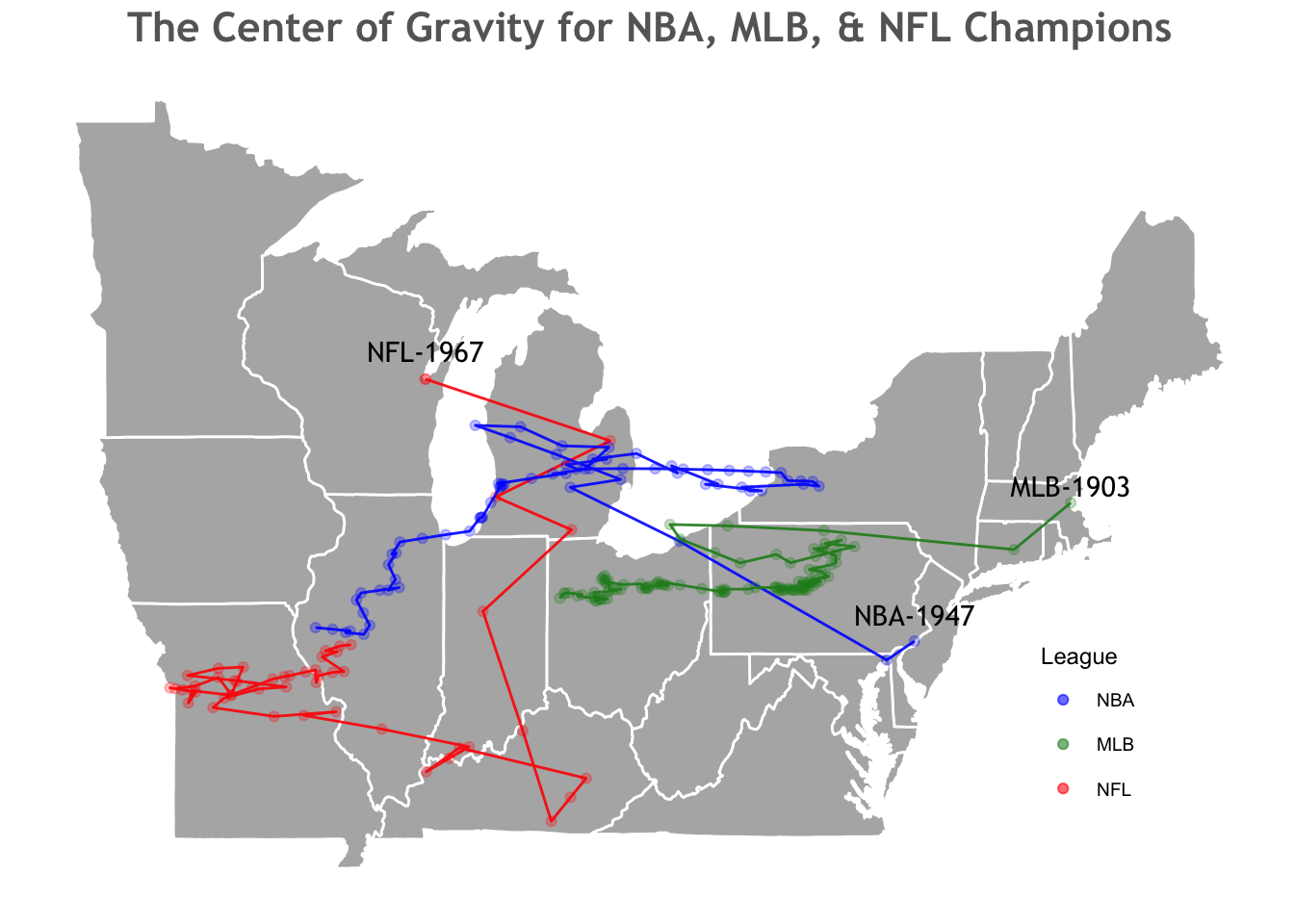Drawing inspiration from FiveThirtyEight’s article locating the Stanley Cup’s center of gravity, or the average geographic point of all Stanley Cup winners, I wondered where other leagues (MLB, NBA, NFL) championship trophies’ geographical center could lie. Using Cartesian coordinates for each champion’s city, I mapped the location for each league. The corresponding code and plots are shown below.

### Load Packages

``````library(rvest)
library(tidyverse)
library(ggmap)
library(DT)
library(leaflet) ``````

### Scrape and Clean Data

``````#scrape NFL table
nfl <- read_html("http://www.espn.com/nfl/superbowl/history/winners") %>%
html_node("table") %>%
html_table()

#remove top two header rows
nfl <- nfl[-c(1:2),]

#remove losing teams
nfl <- nfl %>%
separate(X4, into = "Winner", sep = ",")

#remove winning team score
nfl\$Winner <- gsub('[[:digit:]]+', '', nfl[,"Winner"])

#create vector of team names in column
teams <- c("Jets", "Giants", "Steelers", "Saints", "Packers", "Ravens", "Seahawks", "Patriots", "Broncos", "Eagles")

#remove team names from column and replace ambiguous city names
nfl\$Winner <- gsub(paste0(teams,collapse = "|"),"", nfl\$Winner) %>%
trimws("right") %>%
recode("New York" = "New York, NY", "New England" = "Boston", "Washington" = "Washington, D.C.")

#optain latitude and longitude
nfl_locations <- geocode(nfl\$Winner)
nfl <- bind_cols(nfl, nfl_locations)``````
``````#scrape NBA data
nba <- read_html("https://simple.wikipedia.org/wiki/List_of_NBA_champions") %>%
html_node("table") %>%
html_table()

#clean data
nba <- nba[,1:2] %>%
rename(winner = `Winning team`) %>%
separate(winner, into = "Winner", sep = " ")

#replace ambiguous city names
nba\$Winner <- recode(nba\$Winner,"St." = "St. Louis", "New" = "New York, NY", "Los" = "Los Angeles",   "Golden" = "Oakland", "San" = "San Antonio", "Washington" = "Washington, D.C.")

#optain latitude and longitude
nba_locations <- geocode(nba\$Winner)
nba <- bind_cols(nba, nba_locations)``````
``````#scrape MLB data
mlb <- read_html("http://www.espn.com/mlb/worldseries/history/winners") %>%
html_node("table") %>%
html_table()

#remove, sort, and seperate rows
mlb <- mlb[-c(1:2),] %>%
separate(X2, into = "Winner", sep = " ") %>%
arrange(-row_number())

#replace ambiguous city names
mlb\$Winner <- recode(mlb\$Winner, "St." = "St. Louis", "New" = "New York, NY", "Brooklyn" = "New York, NY", "Los" = "Los Angeles", "Kansas" = "Kansas City", "Florida" = "Miami", "Washington" = "Washington, D.C.", "Arizona" = "Phoenix", "San" = "San Francisco")

#optain latitude and longitude
mlb_locations <- geocode(mlb\$Winner)
mlb <- bind_cols(mlb, mlb_locations)``````

### Calculate Center of Gravity

``````#create function to find average point
find_center <- function(df){

df2 <- df %>%
mutate(rad_lon = df[,"lon"]*pi/180, rad_lat = df[,"lat"]*pi/180) %>%
mutate(X = cos(rad_lat) * cos(rad_lon)) %>%
mutate(Y = cos(rad_lat) * sin(rad_lon)) %>%
mutate(Z = sin(rad_lat)) %>%
summarise(X = mean(X), Y = mean(Y), Z = mean(Z)) %>% #find mean
mutate(Lon = atan2(Y,X), Hyp = sqrt(X*X+Y*Y), Lat = atan2(Z, Hyp)) %>%
select(Lon, Lat) %>%
mutate(Lon = Lon*180/pi, Lat = Lat*180/pi)

return(df2)
}

#locate center of gravity for each league
nfl_center <- find_center(nfl)
nba_center <- find_center(nba)
mlb_center <- find_center(mlb)``````
``````#find center of gravity after each year
for (i in 1:nrow(nfl)) {
nfl\$lon_center[i] <- find_center(nfl[1:i,])[]
nfl\$lat_center[i] <- find_center(nfl[1:i,])[]
}

for (i in 1:nrow(nba)) {
nba\$lon_center[i] <- find_center(nba[1:i,])[]
nba\$lat_center[i] <- find_center(nba[1:i,])[]
}

for (i in 1:nrow(mlb)) {
mlb\$lon_center[i] <- find_center(mlb[1:i,])[]
mlb\$lat_center[i] <- find_center(mlb[1:i,])[]
}``````The NBA and MLB seem to be trending westward while the NFL looks to be boomeranging back east of the Mississippi. With the Yankees’ 27 championships (not to mention the NY Giants 5 titles plus the Mets 2 in addition to the Brooklyn Dodgers’ lone Series win) it’s no surprise that the MLB’s center of gravity is the furthest east. The Boston Celtic’s ’60s dynasty seems to have left its mark on the Larry O’Brien trophy, carrying the midpoint to upstate New York before ultimately trickling down through Illinois. Use the map below to toggle between leagues and a see which cities have won the most hardware.

### Total Titles by City

``````nfl_total <- nfl %>%
group_by(Winner) %>%
select(Winner, lon, lat) %>%
add_tally()

mlb_total <- mlb %>%
group_by(Winner) %>%
select(Winner, lon, lat) %>%
add_tally()

nba_total <- nba %>%
group_by(Winner) %>%
select(Winner, lon, lat) %>%
add_tally()``````